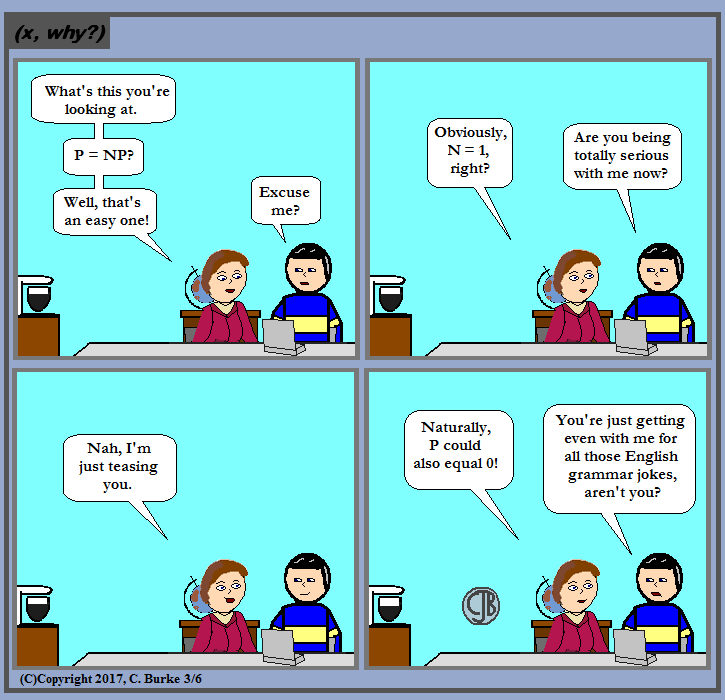## Friday, March 31, 2017

(Click on the comic if you can't see the full image.)They're just two wild and crazy lines.

Come back often for more funny math and geeky comics.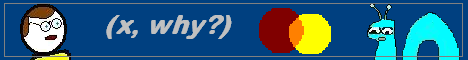## Thursday, March 30, 2017

(Click on the comic if you can't see the full image.)Just watch out for those antioxidants

Come back often for more funny math and geeky comics.## Tuesday, March 28, 2017

### SAS

(Click on the comic if you can't see the full image.)I'd rate Dorothy Hamill higher: her hair was short and SASsy.

Come back often for more funny math and geeky comics.## Monday, March 27, 2017

### Algebra 2 Problems of the Day

The following problems were taken from the ALGEBRA II (Common Core) Regents Exam given on Friday, January 27, 2017.
Previous problems can be found here

### Part 1

9. Gabriel performed an experiment to see if planting 13 tomato plants in black plastic mulch leads to larger tomatoes than if 13 plants are planted without mulch. He observed that the average weight of the tomatoes from tomato plants grown in black plastic mulch was 5 ounces greater than those from the plants planted without mulch. To determine if the observed difference is statistically significant, he rerandomized the tomato groups 100 times to study these random differences in the mean weights. The output of his simulation is summarized in the dotplot below.

Given these results, what is an appropriate inference that can be drawn?

(2) There was an effect observed that could be due to the random assignment of plants to the groups.

10. If p(x) = abx and r(x) = cdx, then p(x) • r(x) equals

(3) ac(bd)x
p(x) • r(x) = abx • cdx = ac • bx • dx = ac • (bd)x

Continue to the next problems

### Geometry Problems of the Day

The following problems were taken from the GEOMETRY (COMMON CORE) Regents Exam given on Thursday, January 26, 2017.
Previous problems can be found here.

### Part 1

9. The diagram shows rectangle ABCD, with diagonal BD

. What is the perimeter of rectangle ABCD, to the nearest tenth?

(2) 32.8.
You could use sin and cos to find the length and the width of the rectangle.
However, the diagonal creates a 30-60-90 right triangle, which has special properties. The side opposite the 30o angle is half the length of the hypotenuse (the diagonal of the rectangle). The side opposite the 60o angle is equal to the shorter side times radical 3, or (3)^(.5).
So the perimeter is 6 + 6 + 6(3)^(.5) + 6(3)^(.5) = 32.7846097, which rounds to 32.8.

10. Identify which sequence of transformations could map pentagon ABCDE onto pentagon A"B"C"D"E", as shown below.

(3) line reflection followed by a translation
Notice the position of B" and D". They indicate that one reflection took place, not a rotation. Likewise, had two reflections taken place, then B" and D" would be in different places.

Continue to the next problems.

## Sunday, March 26, 2017

### Algebra 2 Problems of the Day

The following problems were taken from the ALGEBRA II (Common Core) Regents Exam given on Friday, January 27, 2017.
Previous problems can be found here

### Part 1

7. The expression

is equivalent to

(2) (see below)

You may do some of these steps in a different order. You might combine several of them.
I'm breaking it down as much as I can so more people can follow along.
Here is my process:

Resolve the division by subtracting the exponents: 2 - (1/3) = 1 2/3 or 5/3.
Next resolve the exponents: to find a power or a power, multiply the exponents, so (5/3)(-1/2) = (-5/6)
A negative exponent indicates the reciprocal: m(-5/6) = (1 / m5/6)
A fractional exponent indicates the nth root: see illustration above.

8. What is the inverse of the function y = log 3 x?

(3) y = 3x
Logs are the inverse of exponential functions.

Continue to the next problems

### Geometry Problems of the Day

The following problems were taken from the GEOMETRY (COMMON CORE) Regents Exam given on Thursday, January 26, 2017.
Previous problems can be found here.

### Part 1

7. The diagram below shows two similar triangles.

If tan O = 3/7, what is the value of x, to the nearest tenth?

(2) 5.6.
tan O = 3/7, and tan O = 2.4/x, so 3/7 = 2.4/x.
3x = (7)(2.4) = 16.8
x = 5.6

8. A farmer has 64 feet of fence to enclose a rectangular vegetable garden. Which dimensions would result in the biggest area for this garden?

(1) the length and the width are equal
A dilation increased the size, so it will no longer be congruent.
For all rectangles with the same perimeter, a square will always have the greatest area.
Consider this:
A square with sides equal to x, will have a perimeter of 4x and area of x2.
A rectangle with sides equal to (x+1) and (x-1), will have a perimeter of 4x and area of x2 - 1.
A rectangle with sides equal to (x+2) and (x-2), will have a perimeter of 4x and area of x2 - 4.
A rectangle with sides equal to (x+3) and (x-3), will have a perimeter of 4x and area of x2 - 9.
etc.
The area will always be some perfect subtracted from x2, which will always be less than x2.

Continue to the next problems.

## Saturday, March 25, 2017

### Algebra 2 Regents Problems of the Day

The following problems were taken from the ALGEBRA II (Common Core) Regents Exam given on Friday, January 27, 2017.
Previous problems can be found here

### Part 1

5. What is the solution to the system of equations y = 3x - 2 and y = g(x) where g(x) is defined by the function below?

(4) {(1,1),(6,16)}
First of all, this is an odd question. Three of the answers make no sense at all. It is plain to see that neither (0, -2) nor (1, 6) are points on g(x), the parabola shown.
Second, it is quite plain that (1, 1) is on that line, and that (1) = 3(1) - 2, so it fits the other equation in the system as well. The choice is (4).
Just to be thorough, how do we know that (6, 16) is a solution to g(x)?
You can see that g(x) has points (0, 4), (1, 1) and (2, 0), which is the vertex. This makes (3, 1) and (5, 4) points by reflection over the line of symmetry, if you couldn't tell from the graph. The parent function is x2 and it has been shifted two spaces to the right.
This means that g(x) = (x - 2)2.
Then g(6) = (6 - 2)2 = 16 and (16) = 3(6) - 2.

6. Which statement about statistical analysis is false?

(3) Observational studies can determine cause and effect relationships.
Experiments do that, not observational studies.

Continue to the next problems

### Geometry Regents Problems of the Day

The following problems were taken from the GEOMETRY (COMMON CORE) Regents Exam given on Thursday, January 26, 2017.
Previous problems can be found here.

### Part 1

5. In the diagram below, if triangle ABE = triangle and AEFC is drawn, then it could be proven that quadrilateral ABCD is a

(4) Parallelogram.
First: common sense. If the figure could be proved to be a rectangle or a rhombus, it would have to be a parallelogram as well. You can't have multiple answers. If it were a square, then all four would be true.
Second: figures are not necessarily drawn to scale (even if they don't tell you), so you shouldn't assume that it isn't a square based solely on the picture. Looking at an image isn't proof.

If the triangles are congruent, then by CPCTC (Corresponding parts of congruent triangles are congruent) we know that AB = CD. We also know, for the same reason, that angle BAE = DCF. Those two angles are alternate interior angles along the transversal. That makes AB || CD.
If two sides of a quadrilateral are both parallel and congruent, the shape is a parallelogram.
We do not have any additional information to prove (nor assume!) that the shape is a rhombus.

6. Under which transformation would triangle A'B'C', the image of triangle ABC, not be congruent to ABC?

(4) dilation with a scale factor of 2 centered at the origin.
A dilation increased the size, so it will no longer be congruent.

Continue to the next problems.

## Friday, March 24, 2017

### (x, why?) Mini: Irregular

(Click on the comic if you can't see the full image.)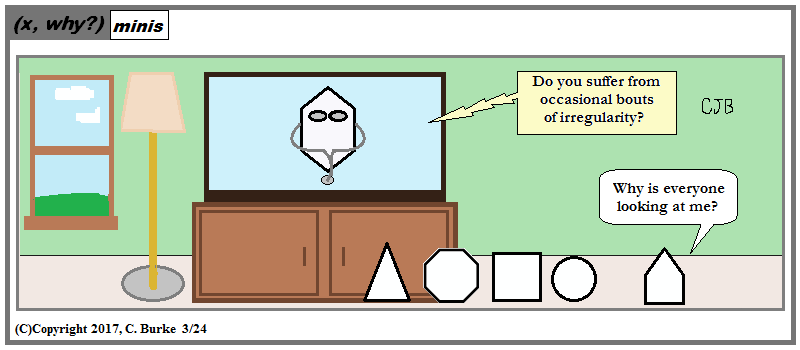To be fair, 3/5ths of the pentagon feels ''right''.

On the other hand, I won't share this with my class because they'll understand the Geometry portion (I hope), but not the doctor on TV part. I'm not explaining it.

Come back often for more funny math and geeky comics.## Thursday, March 23, 2017

(Click on the comic if you can't see the full image.)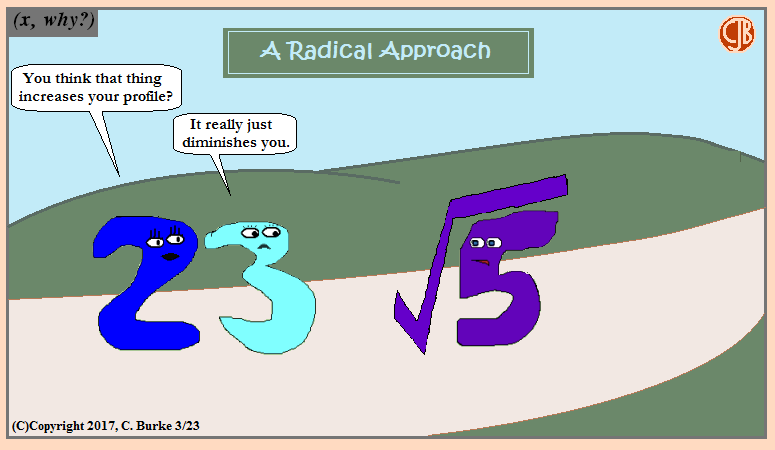He's definitely lower than 3, but he's still more than 2.

Come back often for more funny math and geeky comics.## Wednesday, March 22, 2017

### Algebra 2 Regents Problems of the Day

The following problems were taken from the ALGEBRA II (Common Core) Regents Exam given on Friday, January 27, 2017.
Previous problems can be found here

### Part 1

3. When factored completely, m5 + m3 - 6m is equivalent to

(4) m(m2 + 3)(m2 - 2)
Each term has m, factor it: (m)(m4 + m2 - 6)
Factor into two binomials: What are two factors of -6 that add up to +1: +3 and -2
So it factors into m(m2 + 3)(m2 - 2).

4. If sin2(32°) + cos2(M) = 1, then M equals

(1) 32°.
The rule is: sin2x + cos2x = 1.
In this case x = 32°, so M must be 32° as well.

Continue to the next problems

### Geometry Regents Problems of the Day

The following problems were taken from the GEOMETRY (COMMON CORE) Regents Exam given on Thursday, January 26, 2017.
Previous problems can be found here.

### Part 1

3. Given triangle ABC = triangle DEF, which statement is not always true?(

(1) BC = DF.
BC and DF are not corresponding sides. BC corresponds to EF and DF corresponds to AC. They would only be congruent if the triangles were isosceles or equilateral. We don't know whether they are or not.

4. In the diagram below, DE, DF and EF are midsegments of triangle ABC.

(4) AB + AC.
FE = DB and DE = FC. So AD + DE + EF + FA = AD + FC + DB + FA.
Rearrange the terms: AD + DB + AF + FC = AB + AC.

Continue to the next problems

## Sunday, March 19, 2017

### Algebra 2 Regents Problems of the Day

The following problems were taken from the ALGEBRA II (Common Core) Regents Exam given on Friday, January 27, 2017.

### Part 1

1. Relative to the graph of y = 3sin x, what is the shift of the graph of y = 3sin(x + π/3)?

(2) π/3 left.
When the value within the parentheses is added to x, the graph shifts to the left. When the value within the parentheses is subtracted from x, the graph shifts to the right.
To move up or down, the addition or subtraction would have to take place outside the parantheses.

2. A rabbit population doubles every 4 weeks. There are currently five rabbits in a restricted area. If t represents the time, in weeks, and P(t) is the population of rabbits with respect to time, about how many rabbits will there be in 98 days?

(1) 56.
The starting value is 5. The base is 2. The exponent is 98 days / 4 weeks, which is 98 / 28 after converting weeks to days.
That gives a value of approximately 56.5685424949..., which is approximately 56.
(Yes, that would round up to 57, but that is not one of the choices, leaving 56 as the closest.)

Continue to the next problems

### Geometry Regents Problems of the Day

The following problems were taken from the GEOMETRY (COMMON CORE) Regents Exam given on Thursday, January 26, 2017.

### Part 1

1. Which equation represents the line that passes through the point (-2,2) and is parallel to y = 1/2 x + 8?

(3) y = 1/2 x + 3.
A parallel line will have the same slope, 1/2, so y = 1/2 x + b.
Next, substitute the coordinates (-2, 2) in place of x and y, giving you

2 = 1/2 (-2) + b
2 = -1 + b
3 = b
so y = 1/2 x + 3

2. In the diagram below, triangle ADE is the image of triangle ABC after a reflection over the line AC followed by a dilation of scale factor (AE/AC) centered at point A.

Which statement must be true?

Neither reflection nor dilation changes the shape of the figure, nor the sizes of the angles, and ∠ABC and ∠ADE are corresponding angles in the two triangles.

Continue to the next problems

## Friday, March 17, 2017

### Happy St. Patrick's Day 2017

(Click on the comic if you can't see the full image.)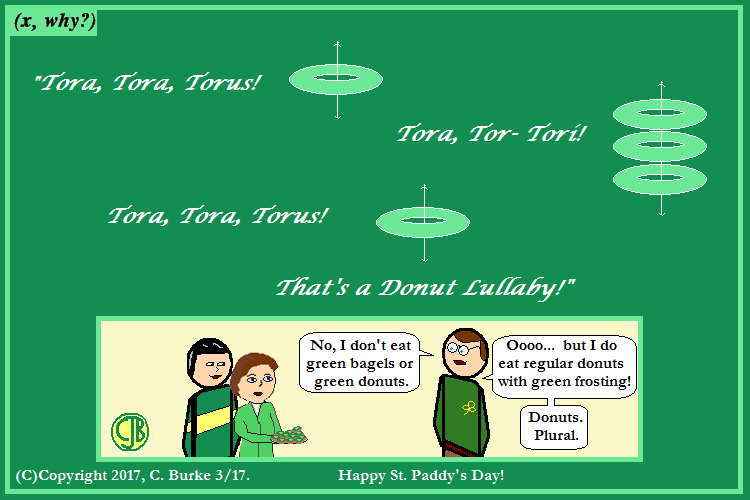Tora Lora Torus just doesn't sound right. But now I might get Pearl Harbor comments

This St. Paddy's Day, may all your non-Irish friends be Green with envy!

Come back often for more funny math and geeky comics.## Wednesday, March 15, 2017

### Sides

(Click on the comic if you can't see the full image.)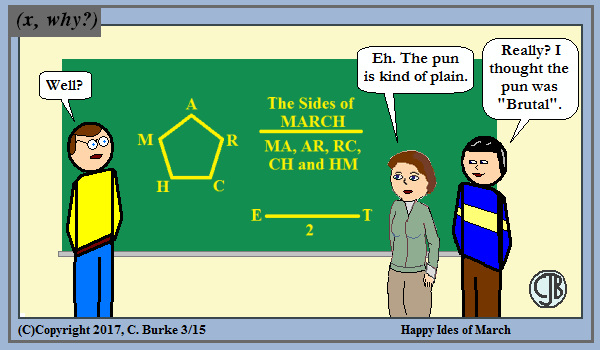Followed by the sound of a dagger striking . . .

Come back often for more funny math and geeky comics.## Tuesday, March 14, 2017

### Pi in the Sky

(Click on the comic if you can't see the full image.)Next Up: Pi on Sports!

I almost went with a family trip on an airline instead. Maybe I should make a note of that for the future.

Come back often for more funny math and geeky comics.## Thursday, March 09, 2017

### (x, why?) Mini: Irreplaceable

(Click on the comic if you can't see the full image.)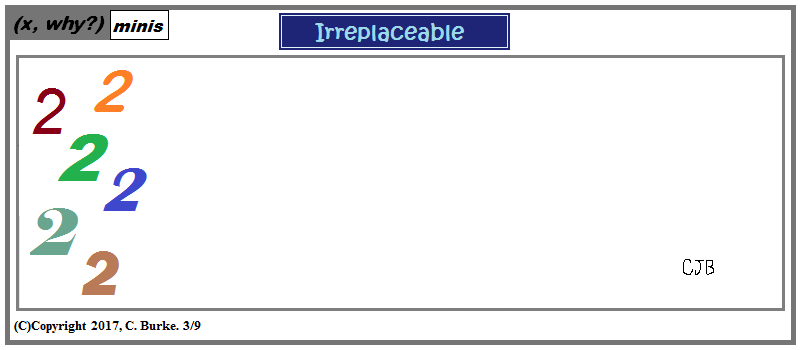Speaks for itself. I hope.

Come back often for more funny math and geeky comics.## Monday, March 06, 2017

### (x, why?) Mini: Improper Fraction

(Click on the comic if you can't see the full image.)He's a bit mixed up.

Come back often for more funny math and geeky comics.### P = NP

(Click on the comic if you can't see the full image.)